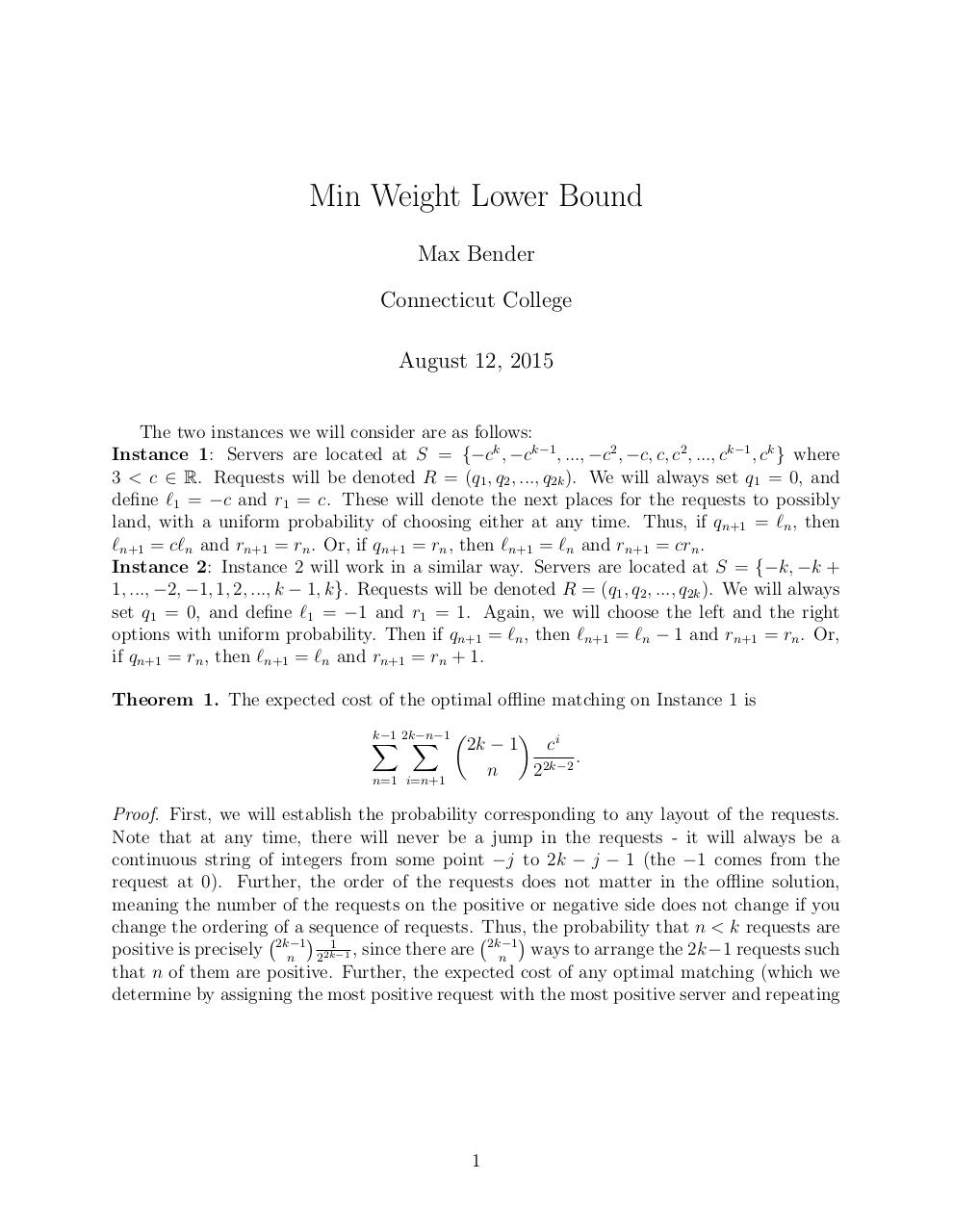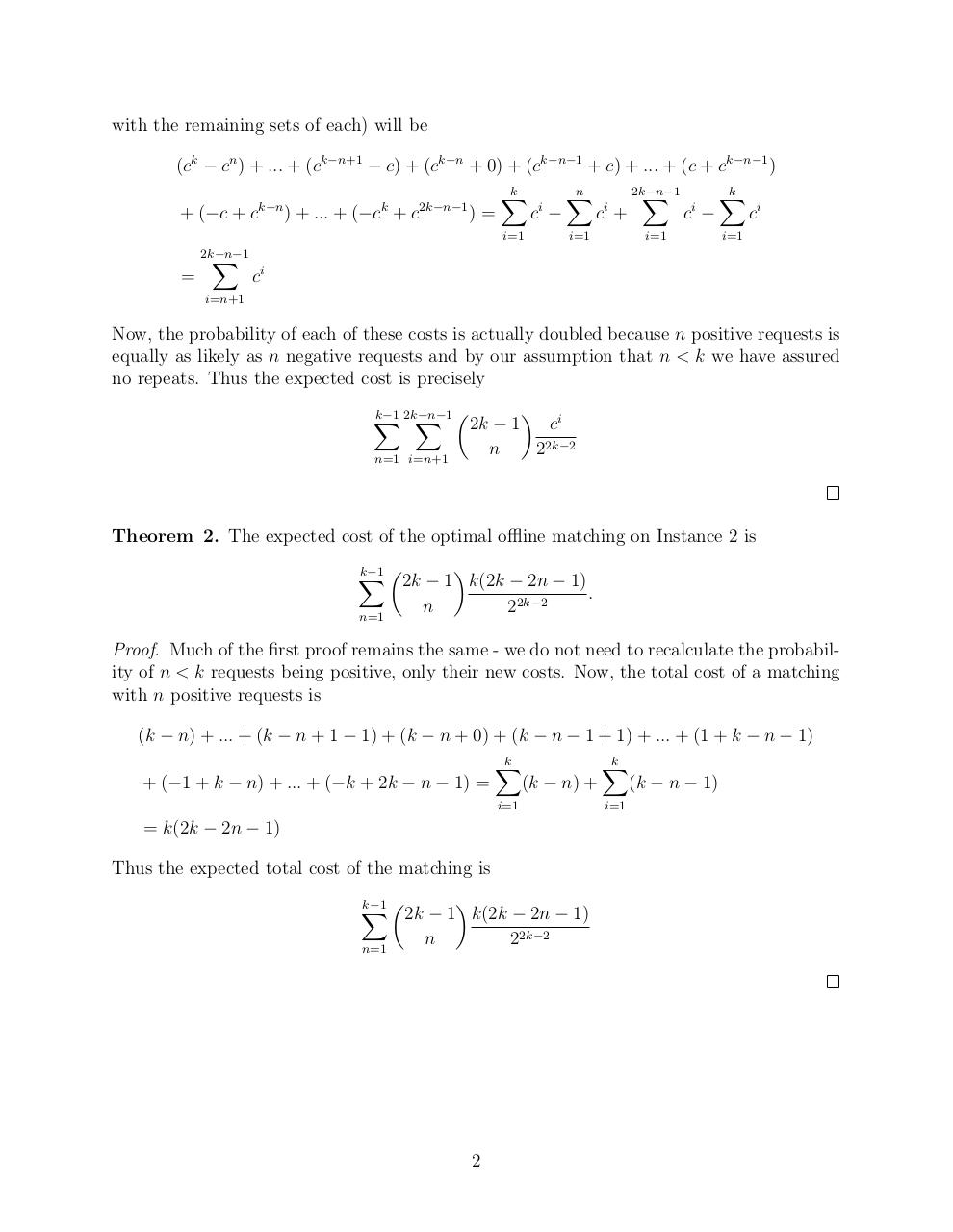# MWLB .pdf

### File information

Original filename: MWLB.pdf

This PDF 1.5 document has been generated by TeX / MiKTeX pdfTeX-1.40.14, and has been sent on pdf-archive.com on 12/08/2015 at 22:04, from IP address 136.244.x.x. The current document download page has been viewed 852 times.
File size: 129 KB (2 pages).
Privacy: public file

MWLB.pdf (PDF, 129 KB)

### Document preview

Min Weight Lower Bound
Max Bender
Connecticut College
August 12, 2015
The two instances we will consider are as follows:
Instance 1: Servers are located at S = {−ck , −ck−1 , ..., −c2 , −c, c, c2 , ..., ck−1 , ck } where
3 &lt; c ∈ R. Requests will be denoted R = (q1 , q2 , ..., q2k ). We will always set q1 = 0, and
define `1 = −c and r1 = c. These will denote the next places for the requests to possibly
land, with a uniform probability of choosing either at any time. Thus, if qn+1 = `n , then
`n+1 = c`n and rn+1 = rn . Or, if qn+1 = rn , then `n+1 = `n and rn+1 = crn .
Instance 2: Instance 2 will work in a similar way. Servers are located at S = {−k, −k +
1, ..., −2, −1, 1, 2, ..., k − 1, k}. Requests will be denoted R = (q1 , q2 , ..., q2k ). We will always
set q1 = 0, and define `1 = −1 and r1 = 1. Again, we will choose the left and the right
options with uniform probability. Then if qn+1 = `n , then `n+1 = `n − 1 and rn+1 = rn . Or,
if qn+1 = rn , then `n+1 = `n and rn+1 = rn + 1.
Theorem 1. The expected cost of the optimal offline matching on Instance 1 is
k−1 2k−n−1
X
X 2k − 1 ci
.
2k−2
2
n
n=1 i=n+1

Proof. First, we will establish the probability corresponding to any layout of the requests.
Note that at any time, there will never be a jump in the requests - it will always be a
continuous string of integers from some point −j to 2k − j − 1 (the −1 comes from the
request at 0). Further, the order of the requests does not matter in the offline solution,
meaning the number of the requests on the positive or negative side does not change if you
change the ordering of a sequence of requests. Thus, the probability that n &lt; k requests are
1
ways to arrange the 2k−1 requests such
positive is precisely 2k−1
, since there are 2k−1
n
n
22k−1
that n of them are positive. Further, the expected cost of any optimal matching (which we
determine by assigning the most positive request with the most positive server and repeating

1

with the remaining sets of each) will be
(ck − cn ) + ... + (ck−n+1 − c) + (ck−n + 0) + (ck−n−1 + c) + ... + (c + ck−n−1 )
+ (−c + c

k−n

k

) + ... + (−c + c

2k−n−1

)=

k
X

i

c −

i=1

=

2k−n−1
X

n
X

i

c +

i=1

2k−n−1
X

i

c −

i=1

k
X

ci

i=1

ci

i=n+1

Now, the probability of each of these costs is actually doubled because n positive requests is
equally as likely as n negative requests and by our assumption that n &lt; k we have assured
no repeats. Thus the expected cost is precisely
k−1 2k−n−1
X
X 2k − 1 ci
22k−2
n
n=1 i=n+1

Theorem 2. The expected cost of the optimal offline matching on Instance 2 is

k−1 
X
2k − 1 k(2k − 2n − 1)
n=1

22k−2

n

.

Proof. Much of the first proof remains the same - we do not need to recalculate the probability of n &lt; k requests being positive, only their new costs. Now, the total cost of a matching
with n positive requests is
(k − n) + ... + (k − n + 1 − 1) + (k − n + 0) + (k − n − 1 + 1) + ... + (1 + k − n − 1)
k
k
X
X
+ (−1 + k − n) + ... + (−k + 2k − n − 1) =
(k − n) +
(k − n − 1)
i=1

= k(2k − 2n − 1)
Thus the expected total cost of the matching is

k−1 
X
2k − 1 k(2k − 2n − 1)
n=1

22k−2

n

2

i=1#### HTML Code

Copy the following HTML code to share your document on a Website or Blog

#### QR Code### Related keywords